# A semi-circular

A semi-circular fishbowl is filled with water and has a diameter of 10 feet. What is the total weigh of water to the nearest pound in the tank, if water weighs 62.5 pounds per cubic foot?

m =  16362 pd

### Step-by-step explanation: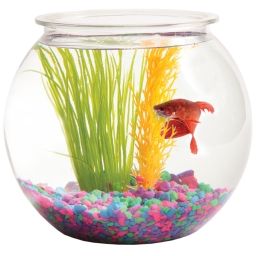Did you find an error or inaccuracy? Feel free to write us. Thank you!Tips to related online calculators
Tip: Our volume units converter will help you with the conversion of volume units.
Tip: Our Density units converter will help you with the conversion of density units.

#### You need to know the following knowledge to solve this word math problem:

We encourage you to watch this tutorial video on this math problem:

## Related math problems and questions:

• The water tankThe water tank has the shape of a sphere with a radius of 2 m. How many liters of water will fit in the tank? How many kilograms of paint do we need to paint the tank, if we paint with 1 kg of paint 10 m2?
• Hemispherical hollowThe vessel hemispherical hollow is filled with water to a height of 10 cm =. How many liters of water are inside if the hollow's inside diameter is d = 28cm?
• Tank diameterA cylindrical tank has a volume of 60 hectoliters and is 2.5 meters deep. Calculate the tank diameter.
• Tank and waterWe poured a cylindrical tank with 3.5 liters of water. If the tank base diameter is 3 dm, how height is the water level in?
• Hollow sphereCalculate the weight of a hollow stříbrné sphere (density 10.5 g/cm3) if the vnitřní diameter is 15 cm and wall thickness is 1 mm.
• Five inlets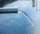The tank can be filled with five equally powerful inlets. If the tank is filled by four of these inlets, it takes a total of 30 minutes to fill one-third of the tank. How many minutes does it take to fill an empty tank if it is filled with all five inlets
• The hemisphereThe hemisphere container is filled with water. What is the radius of the container when 10 liters of water pour from it when tilted 30 degrees?
• Water tankThe water tank has a cylindrical shape with a base diameter of 4.2 m and is 80 cm deep. How many minutes will take fill it 10 cm below the edge of the tank if water flowing 2 liters per second?
• Barrel 2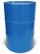Empty barrel weighs 7 kg. When it filled with water up to 45% height, weighs 60 kg. How heavy is barrel full of water?
• Float boya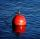A 0.5 meter spherical float is used as a location mark for a fishing boat anchor. It floats in salt water. Find the depth to which the float sinks if the material of which the float is made weighs 8 kilograms per cubic meter and salt water weighs 1027 kg/
• Sphere growthHow many times grow volume of sphere if diameter rises 10×?
• ThereThere is water in the block-shaped tank with dimensions of 3 m 1.5 m 5 m, it occupies 70% of the tank volume. Find the volume of water in the tank (in hl) .
• A residentialA residential colony has a population of 5400, and 60 liters of water is required per person per day. The effective utilization of rainwater constructed a water reservoir measuring 48m × 27m × 25m to collect the rainwater. For how many days, the water of
• Sphere floatingWill float a hollow iron ball with an outer diameter d1 = 20cm and an inside diameter d2 = 19cm in the water? The iron density is 7.8 g/cm3. (Instructions: Calculate the average sphere density and compare it with the water density. )
• Tank B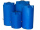Tank B has the same volume as Tank A. The volume of one section of Tank B is 4545 cubic feet. What is the volume, in cubic feet, of the other section of Tank B? Total volume of both tanks A+B is 15000 ft3. Enter your answer in the box.
• Water tank 2Water tank cuboid is 12 meters long and 6.5 meters wide and 1.2 meters high. How many hectoliters are in the tank when it is filled to 81%?
• BallsPing pong balls have a diameter of approximately 5.1 cm. It sold in boxes of 10 pieces: each box has a cuboid shape with a square base. The balls touch the walls of the box. Calculate what portion of the internal volume of the box is filled with balls.